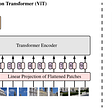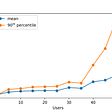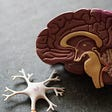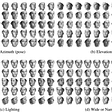# Reading: CNNInvIF / InvIF — Invertibility-Driven Interpolation Filter for Video Coding (HEVC Inter)

## Outperforms FRCNN, 4.7% and 3.6% Average BD-Rate Reduction Under LDB & RA Configurations Respectively

In this story, Invertibility-Driven Interpolation Filter for Video Coding (CNNInvIF / InvIF), by University of Science and Technology of China, Microsoft Research Asia, and University of Missouri-Kansas City, is presented. In this paper:

• Invertibility is introduced: The fractional interpolation filters should not only generate fractional samples from integer samples but also recover the integer samples from the fractional samples in an invertible manner.
• An end-to-end scheme using CNN to train invertibility-driven interpolation filter (InvIF) is proposed.

This is firstly published in 2018 ICIP, then published in 2019 TIP where TIP has a high impact factor of 6.79. In this story, mainly 2019 TIP will be covered. (Sik-Ho Tsang @ Medium)

# Outline

1. Digital Signal Processing: Invertibility
2. CNNInvIF / InvIF: Overall Scheme & Loss Function & Network Architecture
3. HEVC Implementation
4. Experimental Results

# 1. Digital Signal Processing: Invertibility

• Some digital signal processing (DSP) is described here.
• Left: Discrete sampling is performed at integer position:
• Sub-pel position signal can be interpolated based on the discrete sampled signal:
• where M and N are the numbers of samples used for interpolation at the left and right sides, respectively. And is the interpolation filter.
• Right: If we flip the original curve horizontally and the flipped curve becomes s’(t) = s(−t):
• The interpolated value is:
• If there exists an ideal interpolation filter which can perfectly interpolate the fractional samples (i.e. exactly the original analog signal) from the integer samples:
• The integer samples can also be perfectly interpolated from the fractional samples

If there exists a perfect interpolation filter which can recover the fractional samples, then the interpolation filter should also interpolate the integer samples from the fractional samples, due to the duality.

This property is termed invertibility.

• (There are a lot of mathematical proof for this part. Please feel free to read the paper if interested.)

# 2. CNNInvIF / InvIF: Overall Scheme & Loss Function

## 2.1. Overall Scheme

• The proposed scheme consists of two modules: the fractional interpolation module and the invertible reconstruction module.
• An input picture Xi is fed into the scheme, the first InvIF is to generate the fractional-pixel picture Xf. It is noted that the input picture is a compressed version of the original picture.
• The flipping operation T(·) is then performed on the generated fractional-pixel picture Xf. The flipping operation includes horizontal, vertical, and diagonal flipping, according to the target fractional position (xf, yf), 0 ≤ xf, yf<1:
• where xf and yf represent the horizontal and vertical fractional displacements, respectively.
• The flipped picture T(Xf) is then fed into another InvIF followed by the second flipping operation, which aims to recover the original picture X.

The ground truth fractional pixels are synthesized in many prior arts. With the above approach, this issue is solved.

## 2.2. Loss Function

• There are two terms for the loss funciton. The first part is the invertible reconstruction loss:
• where F(·) represents the InvIF illustrated in the above figure.
• Another term is the regularization loss:
• where TIFα(·) isa traditional interpolation function which can lead to the fractional spatial displacement α.
• Bicubic, bilinear and DCTIF are tried. It is found that DCTIF is used which has the best performance.
• This loss is introduced so that the trained interpolation filter is not quite different from a traditional interpolation filter.
• Finally, the total joint loss is:
• where γ is to be 0.5.

## 2.3. Network Architecture

• VRCNN architecture is used.
• VRCNN consists of 4 layers, and in the second and third layers, multi-scale convolutional kernel is utilized.
• Different InvIFs are trained for different QPs to adapt to the variety of video quality.

# 3. HEVC Implementation

• There are two strategies of integrating the proposed InvIF into HEVC, including mode selection between InvIF and DCTIF, and replacing DCTIF with InvIF.
• For the mode selection strategy, a CU-level flag is added.
• For the replacement strategy, DCTIF is simply removed and InvIF is always used for fractional-pixel interpolation.

# 4. Experimental Results

## 4.1. Training

• DIV2K dataset: that consists of 482 pictures with resolution of 2040×1352. 400 pictures are used for training and 82 pictures are used for validation.
• Each training picture is compressed with HEVC intra coding at four QPs (22, 27, 32, 37) to generate the compressed picture.
• HM-16.7 is used under LDB and RA configurations.

## 4.2. BD-Rate

• With InvIF replacing the DCTIF, 2.7% and 2.9% BD-rate reductions are obtained under LDB and RA configurations respectively.
• With InvIF & DCTIF mode selection strategy, 4.7% and 3.6% of larger BD-rate reductions are obtained under LDB and RA configurations respectively.

## 4.2. RD Curves

• The proposed InvIF is not mainly efficient on either low bitrate or high bitrate, but depending on the sequences, as shown above.

## 4.3. Computational Complexity

• It is no doubt that encoder and decoder complexities are high for CNN-based approach.

## 4.4. Selection Ratios

• There are certain amount of CUs choosing InvIF.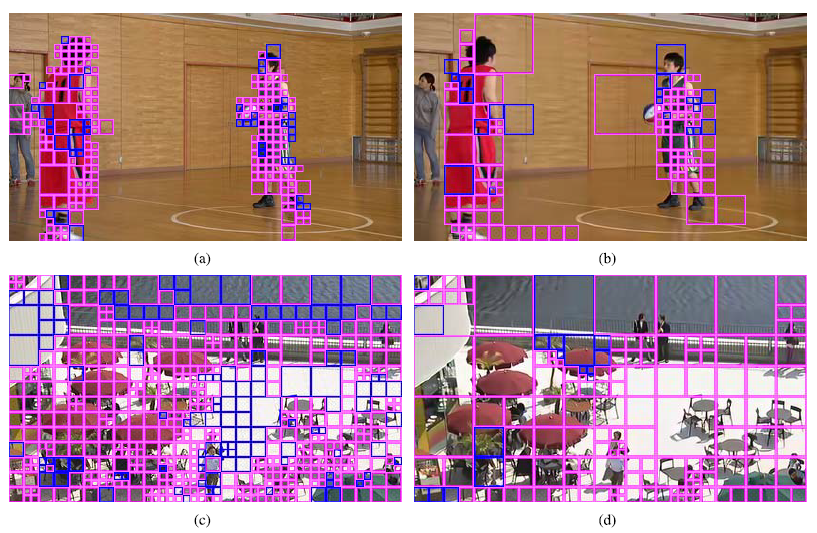pink blocks indicate CUs that choose InvIF, blue blocks indicate CUs that choose DCTIF
• InvIF is preferred at the regions with rich texture, like the clothes and water.

## 4.5. Network Depth

• With deeper network, larger BD-rate reduction can be obtained. But the complexity is also increased largely.

## 4.6. Value of γ

• Different values of γ are tried from 0.2 to 1.
• It is found that γ=0.5 obtains the best performance.

## 4.7 Filters for Regularization Term

• It is found that DCTIF outperforms Bicubic and Bilinear with large margin.

## 4.8 Comparison with FRCNN

• If we simply replace DCTIF with FRCNN, then a great BD-rate increase is observed.
• CU-level selection between DCTIF and FRCNN can bring 2.7% and 1.3% BD-rate reduction for LDB and RA, respectively.

This is the 29th story in this month!

## More from Sik-Ho Tsang

PhD, Researcher. I share what I've learnt and done. :) My LinkedIn: https://www.linkedin.com/in/sh-tsang/, My Paper Reading List: https://bit.ly/33TDhxG

Love podcasts or audiobooks? Learn on the go with our new app.

## Recommended from Medium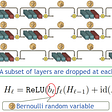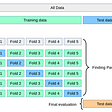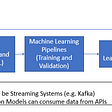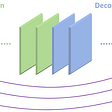## Sik-Ho Tsang

PhD, Researcher. I share what I've learnt and done. :) My LinkedIn: https://www.linkedin.com/in/sh-tsang/, My Paper Reading List: https://bit.ly/33TDhxG

## More from Medium